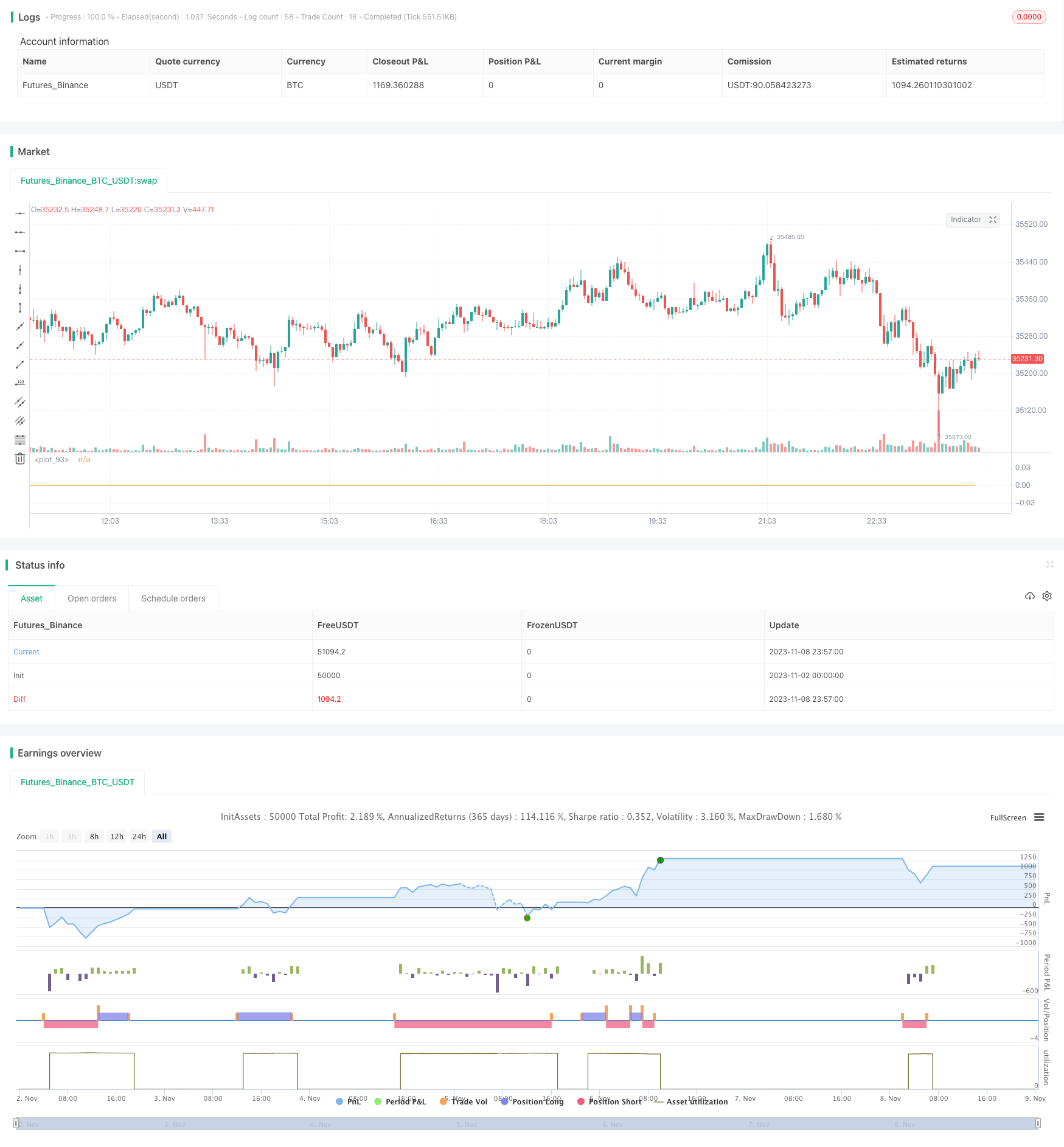# Backtesting and Optimization of RSI Strategy

Author: ChaoZhang, Date: 2023-11-10 11:59:40
Tags:## Overview

This strategy is based on RSI (Relative Strength Index) indicator to determine overbought and oversold conditions. It takes counter trend positions when RSI reaches overbought or oversold levels to buy low and sell high. The strategy is simple and effective, profiting from short-term overbought and oversold scenarios in the market.

## Strategy Logic

The strategy uses RSI indicator solely as the entry signal. It goes long when RSI crosses below the low point (default 20), and goes short when RSI crosses above the high point (default 80). It trades fixed amount each time (default \$100) and aims for 1% profit regardless of market condition. If loss reaches 3%, it stops out. To control trade frequency, strategy stops trading for 24 bars after a losing trade.

The core logic is:

1. Use RSI to determine overbought/oversold
2. Go long when RSI crosses below 20
3. Go short when RSI crosses above 80
4. Trade fixed \$100 each time
5. Take profit or stop loss to close
6. If loss, pause trading for 24 bars

As we can see, the strategy is very simple and mechanical. There is barely any space for parameter optimization. It purely exploits the mathematical properties of RSI to take counter trend positions around overbought/oversold regions.

The biggest advantage of this strategy is simplicity and efficiency.

1. Uses single indicator RSI, no complex technical analysis needed.
2. Fully mechanical system, free from emotional interference.
3. Profits from short-term deviation without predicting market direction.
4. Risk managed with stop loss/take profit.

It also implements stop loss/take profit ratios to lock in profits and control risks, as well as trade suspension to reduce frequency. This maximizes reward while minimizing risks.

## Risk Analysis

Main risks of this strategy come from:

1. Unable to profit in strong trending market. RSI may stay in overbought/oversold zone for extended period when trend persists.

2. Stop loss too wide may lead to excessive loss. Current 3% stop loss may need to be reduced to 1-2%.

4. Fixed \$100 size risks concentration. Should optimize to % of capital.

## Optimization Directions

Based on the analysis, the strategy can be improved in the following ways:

1. Add trend filter like MA to pause trading when trend is unclear.

2. Optimize stop loss/take profit ratios. Reduce stop loss to 1-2% and use dynamic take profit.

3. Limit trade frequency, such as max 2 trades per time period.

4. Size trades based on % of capital instead of fixed \$100.

5. Optimize RSI parameters like period, overbought/sold levels.

6. Add position sizing to not increase size when capital increases.

With these optimizations, the risks can be reduced and stability improved significantly.

## Conclusion

In summary, this is a simple and straightforward strategy using RSI to trade overbought/oversold conditions for short-term mean reversion. The pros are simplicity, efficiency, no prediction needed, clear logic, easy to test. The cons are inability to profit in strong trends and potential losses. With additions like trend filter, optimized parameters, position sizing etc., it can be further enhanced for stability and profitability. The logic is innovative and valuable for practical trading if applied properly.

```/*backtest
start: 2023-11-02 00:00:00
end: 2023-11-09 00:00:00
period: 3m
basePeriod: 1m
exchanges: [{"eid":"Futures_Binance","currency":"BTC_USDT"}]
*/

//@version=5
strategy("rsi超买超卖_回测用", overlay=false, initial_capital=50000, currency=currency.USD, default_qty_type=strategy.cash)
open_pos = input.int(50000, title = "每次开单资金(usdt)")
rsi_period = input.int(14, title = "rsi周期")
rsi_line      = input.float(20.0,      title='RSI触发线',      step=0.05)
stop_rsi_top_line = input.float(70, title = "顶部rsi止损线")
stop_rsi_bottom_line = input.float(30, title = "底部rsi止损线")
stop_loss_perc = input.float(0.03, title = "止损线")
stop_profit = input.float(0.01, title = "止盈")
loss_stop_trade_k = input.int(24, title = "亏损后x根K线不做交易")

rsiParam = ta.rsi(close, rsi_period)

var int failedTimes = 0

// plot(rsiParam)

failedTimes += 1
failedTimes := 0

// 获取当前持仓方向
checkCurrentPosition() =>
strategy.position_size > 0 ? 1 : strategy.position_size < 0 ? -1 : 0

curPosition = checkCurrentPosition()

// 当前持仓成本价
position_avg_price = strategy.position_avg_price

// 当前持单, 触达反向的rsi线，清仓
if curPosition > 0 and rsiParam >= stop_rsi_top_line

if curPosition < 0 and rsiParam <= stop_rsi_bottom_line
strategy.close_all(comment = "closesell")

// 止盈止损清仓
if curPosition > 0
// if (position_avg_price - close) / close >= stop_loss_perc
//     // 止损
if (close - position_avg_price) / position_avg_price >= stop_profit
// 止盈

if curPosition < 0
// if (close - position_avg_price) / position_avg_price >= stop_loss_perc
//     // 止损
//     strategy.close_all(comment = "closesell")

if (position_avg_price - close) / close >= stop_profit
// 止盈
strategy.close_all(comment = "closesell")

var float openPrice = 0.0

if rsiParam <= rsi_line and stopTrade == false
strategy.entry("long", strategy.long, open_pos / close, comment = "long")
if curPosition == 0
openPrice := close
strategy.exit("long_stop", "long", limit = openPrice * (1+stop_profit), stop=openPrice * (1-stop_loss_perc), comment = "closebuy")

if rsiParam >= 100 - rsi_line and stopTrade == false
strategy.entry("short", strategy.short, open_pos / close, comment = "short")
if curPosition == 0
openPrice := close
strategy.exit("short_stop", "short", limit = openPrice * (1-stop_profit), stop=openPrice * (1+stop_loss_perc), comment = "closesell")

plot(failedTimes)
```

More